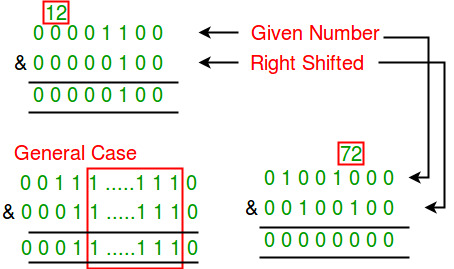# Check if a given number is sparse or not

• Difficulty Level : Medium
• Last Updated : 18 Jan, 2022

A number is said to be a sparse number if in binary representation of the number no two or more consecutive bits are set. Write a function to check if a given number is Sparse or not.
Example :

```Input:  x  = 72
Output: true
Explanation:  Binary representation of 72 is 01001000.
There are no two consecutive 1's in binary representation

Input:  x  = 12
Output: false
Explanation:  Binary representation of 12 is 1100.
Third and fourth bits (from end) are set.```

## We strongly recommend that you click here and practice it, before moving on to the solution.

If we observer carefully, then we can notice that if we can use bitwise AND of binary representation of the “given number its “right shifted number”(i.e., half the given number) to figure out whether the number is sparse or not. Result of AND operator would be 0 if number is sparse and non-zero if not sparse.Below is the implementation of above idea.

## C++

 `// C++ program to check if n is sparse or not` `#include` `using` `namespace` `std;`   `// Return true if n is sparse, else false` `bool` `checkSparse(``int` `n)` `{` `    ``// n is not sparse if there is set` `    ``// in AND of n and n/2` `    ``if` `(n & (n>>1))` `        ``return` `false``;`   `    ``return` `true``;` `}`   `// Driver program` `int` `main()` `{` `    ``cout << checkSparse(72) << endl;` `    ``cout << checkSparse(12) << endl;` `    ``cout << checkSparse(2) << endl;` `    ``cout << checkSparse(3) << endl;` `    ``return` `0;` `}`

## Java

 `// JAVA Code to Check if a` `// given number is sparse or not` `import` `java.util.*;`   `class` `GFG {` `    `  `    ``// Return true if n is` `    ``// sparse,else false` `    ``static` `int` `checkSparse(``int` `n)` `    ``{`   `        ``// n is not sparse if there` `        ``// is set in AND of n and n/2` `        ``if` `((n & (n>>``1``)) >=``1``)` `            ``return` `0``;` `     `  `        ``return` `1``;` `    ``}` `    `  `    ``// Driver code` `    ``public` `static` `void` `main(String[] args)` `    ``{` `        ``System.out.println(checkSparse(``72``)) ;` `        ``System.out.println(checkSparse(``12``)) ;` `        ``System.out.println(checkSparse(``2``)) ;` `        ``System.out.println(checkSparse(``3``)) ;` `        ``}` `    ``}`   `//This code is contributed by Arnav Kr. Mandal.`

## Python3

 `# Python program to check` `# if n is sparse or not`   `# Return true if n is` `# sparse, else false` `def` `checkSparse(n):`   `    ``# n is not sparse if there is set` `    ``# in AND of n and n/2` `    ``if` `(n & (n>>``1``)):` `        ``return` `0` ` `  `    ``return` `1` `    `  `# Driver code` `print``(checkSparse(``72``))` `print``(checkSparse(``12``))` `print``(checkSparse(``2``))` `print``(checkSparse(``30``))`   `# This code is contributed` `# by Anant Agarwal.`

## C#

 `// C# Code to Check if a given` `// number is sparse or not` `using` `System;`   `class` `GFG {` `    `  `    ``// Return true if n is` `    ``// sparse,else false` `    ``static` `int` `checkSparse(``int` `n)` `    ``{`   `        ``// n is not sparse if there` `        ``// is set in AND of n and n/2` `        ``if` `((n & (n >> 1)) >= 1)` `            ``return` `0;` `    `  `        ``return` `1;` `    ``}` `    `  `    ``// Driver code` `    ``public` `static` `void` `Main()` `    ``{` `        ``Console.WriteLine(checkSparse(72));` `        ``Console.WriteLine(checkSparse(12));` `        ``Console.WriteLine(checkSparse(2)); ` `        ``Console.WriteLine(checkSparse(3));` `    ``}` `}`   `// This code is contributed by Sam007.`

## PHP

 `> 1))` `        ``return` `0;`   `    ``return` `1;` `}`   `// Driver Code` `echo` `checkSparse(72), ``"\n"``;` `echo` `checkSparse(12), ``"\n"``;` `echo` `checkSparse(2), ``"\n"``;` `echo` `checkSparse(3), ``"\n"``;`   `// This code is contributed by Ajit.` `?>`

## Javascript

 ``

Output :

```1
0
1
0```

Time Complexity: O(1)

Auxiliary Space: O(1)

Note: Instead of right shift, we could have used left shift also, but left shift might lead to overflow in some cases.